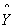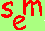David A. Kenny
December 26, 2015
Multiple Regression

View Multiple Regression webinars (small charge or powerpoints (small charge click here)

The example equation:

Y = a + bX + cZ + e

Y   criterion variable
X   predictor variable
a   intercept: the predicted value of Y when all the predictors are zero
b   regression coefficient: how much of a difference in Y results from a one unit difference in X
e   residualpredicted Y given X and Z or equivalently a + bX + cZ (often called "Y hat")
R   multiple correlation: the correlation between Y andThe coefficients (a, b, and c) are chosen so that the sum of squared errors is minimized. The estimation technique is then called least squares or ordinary least squares (OLS). Given the criterion of least squares, the mean of the errors is zero and the errors correlate zero with each predictor.

If the predictor and criterion variables are all standardized, the regression coefficients are called beta weights. A beta weight equals the correlation when there is a single predictor. If there are two or predictors, a beta weights can be larger than +1 or smaller than -1, but this is due to multicollinearity.

The predictors in a regression equation have no order and one cannot be said to enter before the other.

Generally in interpreting a regression equation, it makes no scientific sense to speak of the variance due to a given predictor. Measures of variance depend on the order of entry in step-wise regression and on the correlation between the predictors. Also the semi-partial correlation or unique variance has little interpretative utility.

The standard significance test of whether a specified regression coefficient is equal to zero is to determine if the multiple correlation significantly declines when the predictor variable is removed from the equation and the other predictor variables remain. In most computer programs this is test is given by the t or F next to the coefficient.

Multicollinearity
If two predictors are highly correlated or if one predictor has a large multiple correlation with the other predictors, there is said to be multicollinearity.
With perfect multicollinearity (correlations of plus or minus one), estimation of regression coefficients is impossible. Multicollinearity results in large standard errors for coefficient, and so a statistically significant regression coefficient is difficult (power is low).

Multicollinearity for a given predictor is typically measured by what is called tolerance.  It is defined as 1 - R2 where R2 is the multiple correlation where the predictor now becomes the criterion and the other predictors are the predictors.  Generally tolerance values below .20 are considered potentially problematic.  Another measure is the variance inflation factor which is defined as 1/(1 - R2).  Values above 5 are considered to be potentially problematic.

Suppression
It can occur that a predictor may have little or correlation with the criterion, but have a moderate to large regression coefficient.  For this to happen, two conditions must co-occur:  1) the predictor must be co-linear with one or more other predictor and 2) these predictors have non-trivial coefficients.  With suppression, because the suppressor is correlated with a predictor that has large effect on the criterion, the suppressor should correlate with the criterion.  To explain this, the suppressor is assumed to have an effect that compensates for the lack of correlation.

Diagnostics
It is advisable to examine the errors in the regression equation to determine if they have a normal distribution and that their variance does not change as a function any predictors. Moreover, to evaluate violation of the linearity assumption for a given predictors, one graph the predictor against the errors.

Example
Consider the hypothetical regression equation in which Age (in years) and Gender (1 = Male and -1 = Female) predict weight (in pounds):

Weight =   12 + 22(Gender) + 3(Age) + Error

We interpret the unstandardized coefficients as follows:

intercept: the predicted weight for people who are zero years of age and half way between male and female is 12 pounds
gender: a difference between men and women on the gender variable equals 2 and so there is a 44 (2 times 22) pound difference between the two groups
age: a difference of one year in age results in a difference of 3 pounds

It is advisable to center the Age variable.  To center Age, we would subtract the mean age from Age.  Doing so, would change the intercept to the predicted score for persons of average age in the study.

Note that if we recoded gender to be 1 = Male and 0 = Female, the new equation would be:

Weight =   -10 + 44(Gender) + 3(Age) + Error

intercept: the predicted weight for women who are zero years of age and is ‑10 pounds
gender: men weigh on average  44 more pounds than women, controlling for age
age: a difference of one year in age results in a difference of 3 pounds

Go to the next SEM page.Go to the main SEM page.